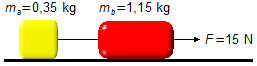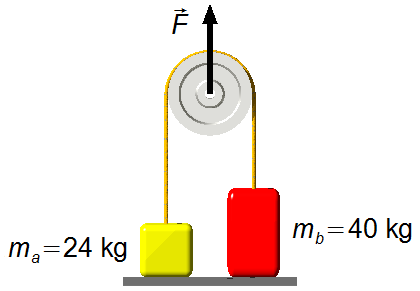Dynamics

### Basic ConceptsTwo blocks, of masses mA = 0.35 kg and mB = 1.15 kg, are on a frictionles horizontal surface, the blocks are connected by a rope with negligible mass. A horizontal force of constant magnitude equal to 15 N is applied by pulling the two blocks. Find the magnitude acceleration acquired by the system and the tension in the rope connecting the blocks.A locomotive, mass 130 t, drags a railroad car, mass 120 t. The maximum force that the locomotive coupler can withstand is 2,900 kN. Find the maximum driving force that the locomotive can exert in order not to break the coupler. Disregard resistance forces.

### Atwood Machine

An Atwood machine has masses, of mA = 6.25 kg and mB = 6.75 kg, connected by a weightless cord, through a pulley frictionless. Find:
a) The acceleration of the system;
b) The tension in the rope connecting the masses;
c) The tension in the rope that holds the system to the ceiling.
Assume the free-fall acceleration g = 10 m/s2.In the system of the figure, the body A slides on a horizontal surface without friction, dragged by body B that moves downward. Bodies A and B are attached by a rope of negligible mass parallel to the surface and passing through a frictionless pulley of negligible mass. The masses of A and B are respectively 32 kg and 8 kg. Find the acceleration of the system and the tension on the cord. Assume g = 10 m/s2.In the system of the figure, the body B slides on a horizontal surface without friction, it is connected through by ropes and pulleys, lightweight and frictionless, with two bodies A and C that move vertically. The masses of A, B, and C are, respectively, 5 kg, 2 kg and 3 kg. Find the acceleration of the system and the tension in the cord. Assume g = 10 m/s2.In an Atwood machine, the two bodies at rest on a horizontal surface, are connected by a cord, of negligible mass, that passes over a frictionless pulley of negligible mass. The masses weight mA = 24 kg and mB = 40 kg and the free-fall acceleration g = 10 m/s2. Find body accelerations when:
a) F = 400 N;
b) F = 720 N;
c) F = 1200 N.### Resistance Forces

On a windless day, a car travels at a constant speed of 72 km/h, the constant associated with the car shape (c) is equal to 0.6 SI units (International System of Units), and the perpendicular area to the direction of motion is 3 m2. Find the magnitude of the air resistance force.

### Forces in Circular Motion

A high-speed train travels a 2500 m radius curve at a speed of 270 km/h, determine:
a) The centrifugal force felt by a passenger of a mass of 70 kg in a wagon;
b) What should be the speed of a car, traveling a 10 m radius curve, so that the passenger of the train felt the same centrifugal force being in the car. Answer in km/h.